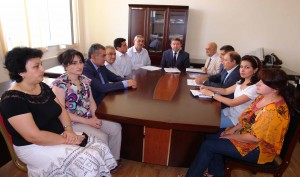﻿ BAKU STATE UNIVERSITY

## The chair of Higher MathematicsThe chair of Higher Mathematics was founded on the basis of the chair of General Mathematics organized in 1997 as common university chair at the faculty of Applied Mathematics and it functioned in the structure of the faculty of Mechanics-Mathematics since 2000.

Prof. A.Kh.Shamilov was the first chief of the chair. Assoc. prof. F.S.Aliyev was the chief of the chair from 2000 till 2002.

At present, the chair has the following staff: Honoured worker of science, d.p.-m.s., prof. M.J.Mardanov (head of the chair), c.p.-m.s., assoc.prof. S.E.Isayeva,, assoc.prof. S.Y.Aliyev, c.p.-m.s., assoc. prof.  M.R.Mehtiyeva, c.p.-m.s., assoc. prof. F.Sh.Mukhtarov, c.p.-m.s., assoc.prof. S.T.Huseynov, lecturer, c.p.-m.s. M.H.Mahmudova, lecturer, c.p.-m.s. G.D.Shukurova.

The chair collaborators are engaged with the questions of optimal control, research of the behavior of solutions of the differential and non-differential equations, inverse and spectral problems of Sturm-Liouville equation, a spectral theory of operator equations, linear singular differential equations in the space of generalized functions, application of theory probability and statistical methods to economical forecasts.

The chair staff takes part in various International Scientific Conferences and Symposiums, their scientific papers have been published in prestigious scientific journals.

The direction of scientific investigations conducted at the chair are study of the questions of existence and uniqueness of solution of some class of initial, boundary and inverse problems for differential equations in partial derivatives and problems of optimal control.

Employments:

Mardanov M.J. - Chief of the chair, professor, doctor of physical and mathematical sciences

Isayeva S.E. - associate professor, candidate of physical and mathematical sciences

Aliyev S.Y. - associate professor, candidate of physical and mathematical sciences

Mehtiyeva M.R. - associate professor, candidate of physical and mathematical sciences

Mukhtarov F.Sh. - associate professor, candidate of physical and mathematical sciences

Huseynov S.T. - associate professor, candidate of physical and mathematical sciences

Shukurova G.D. -

Mahmudova M.H. - sinior lecturer, candidate of physical and mathematical sciences